# 7 Cool Math Proofs Worth Checking Out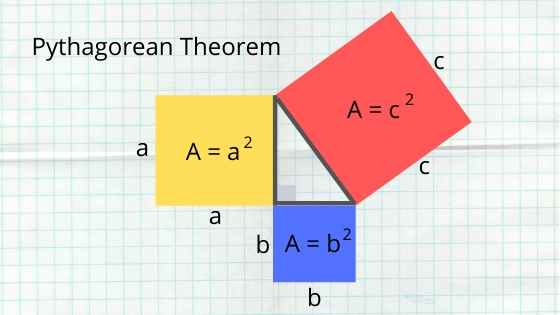Mathematics is a fascinating science where things depend on each other in many different ways and where one quantity can be expressed as the other in order to derive or prove the properties or the very existence of that very quantity.

Here are some simple, yet cool math proofs.

#### Math Proof 1: Prove the Pythagorean Theorem

According to the Pythagorean Theorem the square of two shorter sides in the right angle triangle are equal to the square of the longest side (the hypotenuse).

Visually, this relationship could be represented like this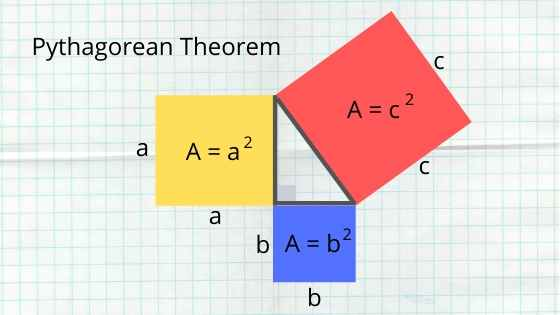To prove that this relationship is true, let’s take a look at the following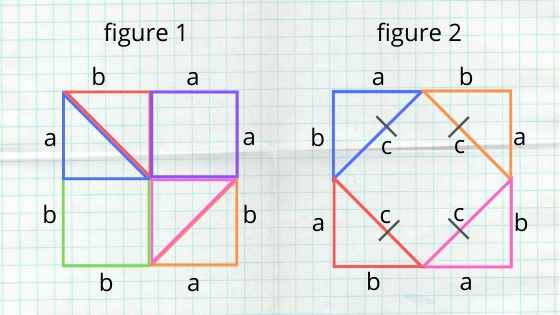Proven.

#### Math Proof 2: Prove that the Area of Triangle is equal to half of the product of its base and height

Here is another one of the cool math proofs.

Given the rectangle, with a shorter side b and a longer side h, we are able to find its area by finding the product the two sides: bh.

Consider two triangles, whose bases are congruent to b and whose heights are congruent to h in the rectangle. One of these triangles will be split into two congruent right angle triangles.

We will get the following:From the diagrams above we can observe that two triangles (placed upside down) whose bases are congruent to b and whose heights are congruent to h in the rectangle together form a rectangle.Proven.

#### Math Proof 3: Prove that the diagonals of a rectangle are congruent

Congruent means equal in size and shape (≅)ABCD is a rectangle, it is also a parallelogram.

Then AB ≌ DC and BC ≌ BC (by the Reflexive Property of Congruence)

⦟ ABC = ⦟ DCB = 90

⦟ ABC ≌ ⦟ DCB since all right angles are congruent

Therefore, AB ≌ DC, BC ≌ BC, ⦟ ABC ≌ ⦟ DCB

so ABC ≌ DCB

and segment AC ≌ segment BD

Proven.

#### Math Proof 4: Prove that the sum of the interior angles in a triangle equals 180 degrees

When two parallel lines are crossed by a transversal, alternate interior angles are equal.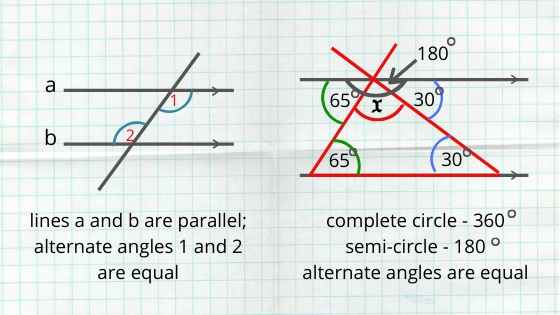Since a complete circle is 360 degrees, then half of it is 180 degrees (semi-circle.)

Therefore, angles in a semi-circle must add up to 180 degrees.

x + 65 + 30 = 180

x = 180 – 95

x = 85o

Then inside the triangle all angles also add up to 180 degrees.

Proven.

#### Math Proof 5: Prove that for all integers n if n is even, then the square of n is even

Let n be any integer. Suppose n is even.

Then n = 2k for some integer k:

n2 = (2k)2 = 4k2 = 2(2k2)

since 2k2 is an integer, n2 is even.

Proven.

#### Math Proof 6: Prove that the sum of the first 100 consecutive whole numbers is 5,050

We can, of course, take every single whole number from 1 to 100 and add it to the previous one. But it will take us a very long time, especially without a calculator.

Instead, if “fold” the numbers and add each pair, we will get a constant sum of 101 (see below). Since there are 100 numbers total, there are 50 pairs of numbers.Thus, the sum of all 100 numbers is 50 x 101 = 5,050

Proven.

#### Math Proof 7: Prove that the area of a circle is the product of Pi and the square of the radius of a circle

A circle can be split into triangles (almost, because there is still a bit of a round portion left over), whose vertices meet at the center of a circle – the more triangles we split it into, the closer the total length of their bases is to that of a circumference of a circle.

Let’s split our circle into 8 triangles for visual purposes and arrange them in a rectangular formation. We almost get a rectangle.

We know that the area of a rectangle is equal to “length × width”. The width of our rectangle will be the same as the radius. To find the length we will need the circumference of the circle.

Since the circumference is equal to 2πr, the base has to be πr.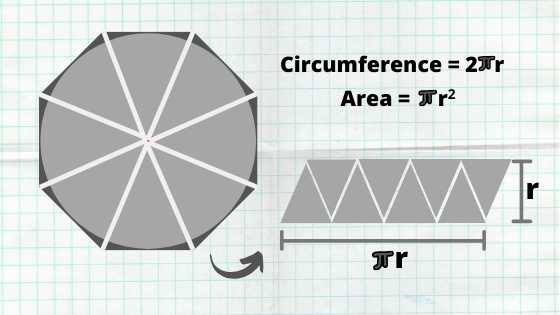Now “length x width” becomes “πr x r”. That gives us πr² which is equal to the area of the rectangle which is equal to the area of the circle.

Proven.

Thanks for checking out our blog.

We are in the Top 100 2019 Math Blogs according to Feedspot!

0